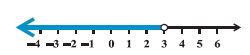Name: ___________________Date:___________________

 Example 1: Solve 7x+3 < 5x+9 Solution: We have 7x+3 < 5x+9 2x < 6 or x < 3Example 2: The marks obtained by a student in his exams are 62 and 48. What marks should he get in his next test to get an average of 60? Solution: (62+8+x)/3 > = 60 x > = 70 Answer: The student must obtain a minimum of 70 marks to get an average of 60.Name: ___________________Date:___________________